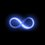# INTrig3GRAL

$\int \frac{ \tan^3 x } { \sin^3 x + \cos^3 x } \, dx$

If you give up, you can look below.5 years, 3 months ago

This discussion board is a place to discuss our Daily Challenges and the math and science related to those challenges. Explanations are more than just a solution — they should explain the steps and thinking strategies that you used to obtain the solution. Comments should further the discussion of math and science.

When posting on Brilliant:

• Use the emojis to react to an explanation, whether you're congratulating a job well done , or just really confused .
• Ask specific questions about the challenge or the steps in somebody's explanation. Well-posed questions can add a lot to the discussion, but posting "I don't understand!" doesn't help anyone.
• Try to contribute something new to the discussion, whether it is an extension, generalization or other idea related to the challenge.

MarkdownAppears as
*italics* or _italics_ italics
**bold** or __bold__ bold
- bulleted- list
• bulleted
• list
1. numbered2. list
1. numbered
2. list
Note: you must add a full line of space before and after lists for them to show up correctly
paragraph 1paragraph 2

paragraph 1

paragraph 2

[example link](https://brilliant.org)example link
> This is a quote
This is a quote
    # I indented these lines
# 4 spaces, and now they show
# up as a code block.

print "hello world"
# I indented these lines
# 4 spaces, and now they show
# up as a code block.

print "hello world"
MathAppears as
Remember to wrap math in $$ ... $$ or $ ... $ to ensure proper formatting.
2 \times 3 $2 \times 3$
2^{34} $2^{34}$
a_{i-1} $a_{i-1}$
\frac{2}{3} $\frac{2}{3}$
\sqrt{2} $\sqrt{2}$
\sum_{i=1}^3 $\sum_{i=1}^3$
\sin \theta $\sin \theta$
\boxed{123} $\boxed{123}$

Sort by:

$\displaystyle \int \frac{\tan^3 x}{\sin^3 x +\cos^3 x} dx= \int \frac{\tan^3 x +1}{\sin^3 x +\cos^3 x} dx - \int \frac{1}{\sin^3 x +\cos^3 x} dx$

Now working on the first one, take $\displaystyle \cos^3 x$ as a common factor in the denominator : $\displaystyle \int \frac{\tan^3 x +1}{\sin^3 x +\cos^3 x} dx = \int \frac{\tan^3 x +1}{\cos^3 x (\tan^3 x +1)} dx = \int \sec^3 x dx$

Let $\displaystyle I = \int \sec^3 x dx$ , Integrating by parts with $\displaystyle u=\sec x , dv= \sec^2 x dx$ :

$\displaystyle I= \sec x \tan x - \int \sec x \tan^2 x dx= \sec x \tan x - \int \sec x (\sec^2 x -1) dx = \sec x \tan x - \int \sec^3 x dx + \int \sec x dx$

$\displaystyle => I= \sec x \tan x - I + \ln(\sec x + \tan x)$

$\displaystyle => I= \frac{ \sec x \tan x + \ln(\sec x + \tan x)}{2}$

Then we have :

$\displaystyle \int \frac{\tan^3 x}{\sin^3 x +\cos^3 x} dx = \frac{ \sec x \tan x + \ln(\sec x + \tan x)}{2} - \int \frac{1}{\sin^3 x +\cos^3 x} dx$

You can see the solution to the last integral from This note. Thus:

$\displaystyle \boxed{\int \frac{\tan^3 x}{\sin^3 x +\cos^3 x} dx = \frac{ \sec x \tan x + \ln(\sec x + \tan x)}{2} - \frac{\sqrt{2}}{6} \ln(\frac{\cos x -\sin x -\sqrt{2}}{\cos x -\sin x +\sqrt{2}}) + \frac{2}{3} \arctan(\cos x -\sin x ) + C}$

- 5 years, 3 months ago

how did you type the integral sign

- 5 years, 2 months ago

\displaystyle \int

between $and$

- 5 years, 2 months ago

Ahha very tricky! I see what you did there. Very good.

Hmm... Which one looks more threatening, one with tangent or one with just sin and cos on bottom? Sayin' cause it seems like tan can be used to simplify sin and cos, and plus when I usually see many things I think they can be manipulated in a certain way to be less complex (like this). And when there's less stuff it seems like you have less options to work with and it all looks scary lol... But this is cause I'm experienced, I guess. What about for those who ain't that pro at math? DUnno... Lol.

Well, King Integral, I've got more stuff coming up for ya. Meanwhile, maintain that streak (we're head to head)! Whoever breaks first is a dweeb.

Now get back to being awesomesalsa

- 5 years, 3 months ago

Yes u are right , integrals with less expressions are more confusing more threatening.

Btw I will try to keep my streak alive although my school began today :)

- 5 years, 3 months ago

Hehe well I kinda cheat: go to practice, algebra, pattern recognition. takes 5 seconds xD

the toughest part is not to forget. but yeh, go with that if ya want :D

- 5 years, 3 months ago

Cheating huh?!

heheh me too I solve level one algeba problem to keep my streak :))

- 5 years, 3 months ago

O_O oooomg no way did you actually do this?? I got no time to check but WHOA and for some reason your answer looks waay shorter than and of WolframAlpha's answers. I'ma check later but great job if you got it right

- 5 years, 3 months ago

yes I checked it several times,also I will try to find a way to prove that wolfram alpha answers equal this.

- 5 years, 3 months ago

Heh if you do that that'd be totally on hook. Also try to do it without like just differentiating it, that's kinda wack.

right now me gotta speed off somewhere, but I shall address this feat when I return. Think of any cool names for yourself by then ;) I thought of Intergral Master or King Integral lol...

- 5 years, 3 months ago

Hehehehe :)

- 5 years, 3 months ago

here ya go:s

And yeah this is doable...sa

Whoever solves this gets to call itself whatever it wants.

SOLVE ON

Here's some extra booze if you need some:aga

- 5 years, 3 months ago